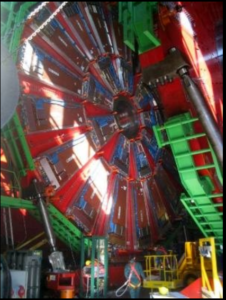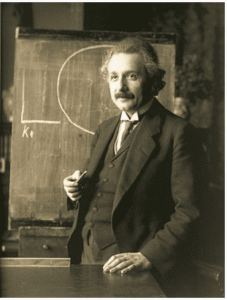Home » Frequently Asked Questions (FAQ) » What is the difference between theoretical quantum physics and experimental quantum physics?

# What is the difference between theoretical quantum physics and experimental quantum physics?

Short answer: Theoretical physics is one of two branches of physics: theoretical and experimental. Like other types of physics, quantum physics has both a theoretical physics branch and an experimental physics branch.

Explanation: Experimental physicists do experiments to find out how accurately physics theories describe the real world. For example, they shoot atoms and bits of atoms around in particle accelerators. They measure the speed of light when it travels through water versus empty space, and so on.Particle accelerator at CERN in Switzerland [Source: By User:Freerk – Own work, CC BY-SA 3.0, https://commons.wikimedia.org/w/index.php?curid=455724] Theoretical physicists don’t do experiments. They read articles and books about physics experiments. They think. And they play with a lot of mathematical equations on black boards or white boards. Then, if these equations accord with experimental results, they publish them along with explanatory text in physics journals.Albert Einstein at the blackboard, 1921 [Source: https://en.wikipedia.org/wiki/Albert_Einstein; in Public Domain]The job of theoretical physicists is to develop mathematical equations and verbal explanations that describe the results of physics experiments. Ideally, theoretical physicists also use their explanations and mathematical equations to predict experimental results. Albert Einstein and Stephen Hawking were both theoretical physicists. They did not do experiments. They studied, thought, and developed new theories and equations.

Now to turn to quantum physics. Quantum physics is the field of physics in which physicists learn about certain types of unusual behavior of particles smaller than atoms but sometimes the size of atoms or even larger. They focus on the “quantum state.” This is the state in which these tiny particles can be in many positions at the same time and where they can act as both waves and particles. Many quantum physicists are theoretical physicists. But many others do experiments.

This is the same in most other fields of physics. For example, physicists who study the physics of heavenly bodies, astrophysicists, can be either theoreticians or experimentalists. The experimentalists often use telescopes for their work. The theoreticians write equations, these days, on white boards.

Before the mid-1900’s, most physicists were both experimentalists and theoreticians. For example, Isaac Newton, who founded modern physics, did many famous experiments. For example, he shot beams of light through prisms. But he also was one of the inventors of calculus, which he used when developing theories which explained the results of his experiments on the motion of objects. Isaac Newton developed the modern type of physics theory—ones which are expressed using mathematical equations. As an example of a mathematical theory, Einstein’s equation, e = mc2, is his theory of the relationship between the quantity of energy and of mass.

Today, few, if any, physicists do both theoretical and experimental work. Both fields require extremely specialized knowledge and skills. But theoreticians and experimentalists work closely with each other to push physics forward.

This site uses Akismet to reduce spam. Learn how your comment data is processed.